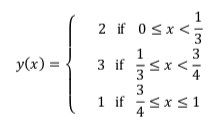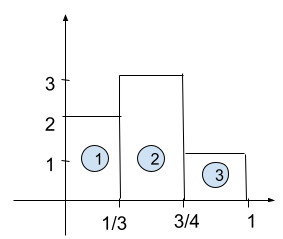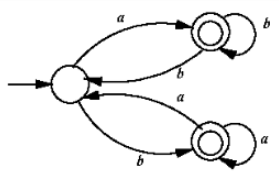## GATE 2022

 Question 1
The _________ is too high for it to be considered _________.
 A fair / fare B faer / fair C fare / fare D fare / fair
Aptitude       English       Video-Explanation
Question 1 Explanation:
Fare: The money paid for a journey. Fair: treating people equally without favouritism or discrimination (or) without cheating (or) trying to achieve unjust advantage The fare is too high for it to be considered fair.
 Question 2
A function y(x) is defined in the interval [0, 1] on the x-axis asWhich one of the following is the area under the curve for the interval [0, 1] on the x-axis?
 A 5/6 B 6/5 C 13/6 D 6/13
Aptitude       Numerical       Video-Explanation
Question 2 Explanation:We know that area of a rectangle = length * breadth = L * B
Area of rectangle (1) = L*B = (1/3) * 2 = (2/3)
Area of rectangle (2) = L*B = (3/4 - 1/3 ) * 3 = (5/4 )
Area of rectangle (3) = L*B = (1 - 3/4) * 1 = 1/4
Therefore Total area = Area(1+2+3) = (2/3) + (5/4) + (1/4) = 26/12 = 13/6
 Question 3
Let r be a root of the equation x2 + 2x + 6 = 0. Then the value of the expression (r + 2)(r + 3)(r + 4)(r + 5) is
 A 51 B -51 C 126 D -126
Aptitude       Numerical       Video-Explanation
Question 3 Explanation:Question 4
Given below are four statements.
Statement 1: All students are inquisitive.
Statement 2: Some students are inquisitive.
Statement 3: No student is inquisitive.
Statement 4: Some students are not inquisitive.
From the given four statements, find the two statements that CANNOT BE TRUE simultaneously, assuming that there is at least one student in the class.
 A Statement 1 and Statement 3 B Statement 1 and Statement 2 C Statement 2 and Statement 4 D Statement 3 and Statement 4
Aptitude       Reasoning       Video-Explanation
Question 4 Explanation:
Explanation: Statement 1 & Statement 3 cannot be true simultaneously.
Statement 1: All students are inquisitive. Statement 3: No student is inquisitiveQuestion 5
A palindrome is a word that reads the same forwards and backwards. In a game of words, a player has the following two plates painted with letters.From the additional plates given in the options, which one of the combinations of additional plates would allow the player to construct a five-letter palindrome. The player should use all the five plates exactly once. The plates can be rotated in their plane.
 ABCDAptitude       Numerical       Video-Explanation
Question 5 Explanation:
From the question we know that letters can rotate in the plane, now we will verify the options one by one. Option 1: A,D,D,D,J from these letters we can't form a palindrome.
Option 2: A,D,R,A,R from these we can form RADAR which is a palindrome.
Option 3: A,D,Z,E,D from these letters we can't form a palindrome.
Option 4: A,D,I,L,Y from these letters we can't form a palindrome.
 Question 6
Some people believe that “what gets measured, improves”.
Some others believe that “what gets measured, gets gamed”.
One possible reason for the difference in the beliefs is the work culture in organizations. In organizations with good work culture, metrics help improve outcomes.
However, the same metrics are counterproductive in organizations with poor work culture.
Which one of the following is the CORRECT logical inference based on the information in the above passage?
 A Metrics are useful in organizations with poor work culture B Metrics are useful in organizations with good work culture C Metrics are always counterproductive in organizations with good work culture D Metrics are never useful in organizations with good work culture
Aptitude       Reasoning       Video-Explanation
Question 6 Explanation:
From the given passage consider the sentence “In organizations with good work culture, metrics help improve outcomes” Option A: Metrics are useful in organizations with poor work culture. Which is incorrect based on the sentence.
Option B: Metrics are useful in organizations with good work culture. Which is correct and relevant to the considered sentence.
Option C: Metrics are always counterproductive in organizations with good work culture. Which is incorrect because we can't always guarantee good work culture.
Option D: Metrics are never useful in organizations with a good work culture. Incorrect metrics will help to make a good work culture.
 Question 7
In a recently conducted national entrance test, boys constituted 65% of those who appeared for the test. Girls constituted the remaining candidates and they accounted for 60% of the qualified candidates. Which one of the following is the correct logical inference based on the information provided in the above passage?
 A Equal number of boys and girls qualified B Equal number of boys and girls appeared for the test C The number of boys who appeared for the test is less than the number of girls who appeared D The number of boys who qualified the test is less than the number of girls who Qualified
Aptitude       Numerical       Video-Explanation
Question 7 Explanation:
The girls accounted for 60% of the qualified candidates.
60% can be written as ⅗. Which means among 5 qualified persons three of them are girls.
So, The number of boys qualified is less than the number of girls qualified.
 Question 8
A box contains five balls of same size and shape. Three of them are green coloured balls and two of them are orange coloured balls. Balls are drawn from the box one at a time. If a green ball is drawn, it is not replaced. If an orange ball is drawn, it is replaced with another orange ball. First ball is drawn. What is the probability of getting an orange ball in the next draw?
 A 1/2 B 8/25 C 19/50 D 23/50
Aptitude       Reasoning       Video-Explanation
Question 8 Explanation:
Case 1: The first ball is Green
Probability of first ball is green = 3/5
If the first ball is green then we are not replacing so the probability of the second ball being orange is = 2/4.
Probability of first ball green and second ball orange is =3/5 * 2/4 = 6/20
Case 2: The first ball is orange
Probability of first ball is orange =2/5
If the first ball is orange then we are replacing it with another orange ball/ so the number balls will remain the same.
Probability of second ball is orange = 2/5
Probability of first ball is orange and second ball is also orange = 2/5*2/5 = 4/25
Therefore, Total probability = (6/20 + 4/25) = 23/50.
 Question 9
The corners and mid-points of the sides of a triangle are named using the distinct letters P, Q, R, S, T and U, but not necessarily in the same order. Consider the following statements:
The line joining P and R is parallel to the line joining Q and S.
P is placed on the side opposite to the corner T.
S and U cannot be placed on the same side.
Which one of the following statements is correct based on the above information?
 A P cannot be placed at a corner B S cannot be placed at a corner C U cannot be placed at a mid-point D R cannot be placed at a corner
Aptitude       Reasoning       Video-Explanation
Question 9 Explanation:
From the given information draw the image, such thatThe Image is satisfying all given conditions. From this we can say that S cannot be placed at the corner. By satisfying all given conditions we can draw in different ways but in all cases S cannot be placed at the corner.
 Question 10
A plot of land must be divided between four families. They want their individual plots to be similar in shape, not necessarily equal in area. The land has equally spaced poles, marked as dots in the below figure. Two ropes, R1 and R2, are already present and cannot be moved.
What is the least number of additional straight ropes needed to create the desired plots? A single rope can pass through three poles that are aligned in a straight line.A 2 B 4 C 5 D 3
Aptitude       Numerical       Video-Explanation
Question 10 Explanation:
Given Conditions are Shape is to be similar, area need not to be same, A rope can pass through three poles.Therefore least number of additional ropes required = 3.
 Question 11
Which one of the following statements is TRUE for all positive functions f (n) ?
 A f ( n ^2 ) ( f ( n ) ^2 ), when f ( n ) is a polynomial B f ( n ^2 ) o ( f ( n ) ^2 ) C f ( n ^2 ) O ( f ( n ) ^2 ), when f ( n ) is an exponential function DAlgorithms       Asymptotic-Complexity       Video-Explanation
Question 11 Explanation:
constant < logarithmic < linear < polynomial < exponential < factorial
f(n)=n^c where c is a constant
f(n^2) = (n^2)^c = n^2c
(f(n))^2 = (n^c)^2 = n^2c
f(n^2) = (f(n))^2 is TRUE asymptotically.
Option-B: FALSE: The small omega function indicates the tightest upper bound.
f(n)^2 < o(f(n)^2) is FALSE asymptotically.
Option-C: FALSE: Consider f(n)=logn
f(n^2)=logn^2 = 2*logn
(f(n))^2 = (logn)^2 = logn * logn
f(n^2) <=Ω(f(n))^2 is FALSE asymptotically.
Option-D: FALSE:
Exponential values(Eg: 2^n, 3^n,..,k^n where k is a constant ”
f(n)=3^n
f(n^2)=f(3^(n^2)
(f(n))^2 = (3^n)^2 = 3^2n
f(n^2) >= O(f(n))^2 is FALSE asymptotically.
 Question 12
Which one of the following regular expressions correctly represents the language of the finite automaton given below?A ab*bab* + ba*aba* B (ab*b)*ab* + (ba*a)*ba* C (ab*b + ba*a)* (a* + b*) D (ba*a + ab*b)* (ab* + ba*)
Theory-of-Computation       Regular-Expression       Video-Explanation
Question 12 Explanation:
Regular expression ab*bab* +ba*aba* does not generate the string “abb” which is accepted by FA hence this is not true.
(ab*b)*ab*+(ba*a)*ba* does not generate the string “abbaa” hence this is also wrong.
(ab*b+ba*a)*(a*+b*) generates epsilon hence this is also wrong.
(ab*b+ba*a)*(ab*+ba*) is the correct regular expression.
GATE 2022 Computer Science and Information Technology (CS)
 Question 13
Which one of the following statements is TRUE?
 A The LALR (1) parser for a grammar G cannot have reduce-reduce conflict if the LR (1) parser for G does not have reduce-reduce conflict. B Symbol table is accessed only during the lexical analysis phase. C Data flow analysis is necessary for run-time memory management. D LR (1) parsing is sufficient for deterministic context-free languages.
Compiler-Design       Parsers       Video-Explanation
Question 13 Explanation:
Even though there is no reduce-reduce conflict in CLR(1) but in LALR(1) while merging the states differ in only lookahead may get reduce-reduce conflict. So the given statement is not true.
Symbol table is accessed in all the phases of compiler and not only in lexical analysis phase.
Data flow analysis is done in the control flow graph, in the code optimization phase. If LR(1) parses a grammar then definitely it is DCFL, so LR(1) parsing is sufficient for deterministic context-free languages.
 Question 14
In a relational data model, which one of the following statements is TRUE?
 A A relation with only two attributes is always in BCNF. B If all attributes of a relation are prime attributes, then the relation is in BCNF. C Every relation has at least one non-prime attribute. D BCNF decompositions preserve functional dependencies.
Database-Management-System       Normalization       Video-Explanation
Question 14 Explanation:
A relation with only two attributes will always be in BCNF.
Example:
R(A, B).
Two functional dependencies possible for the relation: (1) A->B and (2) B->A
If there is no functional dependency, we can assume trivial functional dependencies like AB->A and AB->B.
In all cases, functional dependencies like A->B, A must be a key.
So they all will be in BCNF irrespective of the functional depencies set.
 Question 15
Consider the problem of reversing a singly linked list. To take an example, given the linked list below,Which one of the following statements is TRUE about the time complexity of algorithms that solve the above problem in O (1) space?
 ABCD It is not possible to reverse a singly linked list in O (1) space.
Question 15 Explanation:
struct Node {
int data;
struct Node* next;
{
this->data = data;
next = NULL;
}
};
/* Function to reverse the linked list */
void reverse()
{
// Initialize current, previous and
// next pointers
Node *prev = NULL, *next = NULL;
while (current != NULL) {
// Store next
next = current->next;
// Reverse current node's pointer
current->next = prev;
// Move pointers one position ahead.
prev = current;
current = next;
}
}
For the above algorithm :
Time Complexity: O(n)
Space Complexity: O(1)
 Question 16
Suppose we are given n keys, m hash table slots, and two simple uniform hash functions h 1 and h 2 . Further suppose our hashing scheme uses h 1 for the odd keys and h 2 for the even keys. What is the expected number of keys in a slot?
 A m/n B n/m C 2n/m D n/2m
Algorithms       Hashing       Video-Explanation
Question 16 Explanation:
For n keys and m elements without any condition the expected number of elements in a slot are n/m.
How?
For 1st element the probability of key1 ends up in slot 1 is 1/m.
For 2nd element the probability of key2 ends up in slot 2 is 1/m
..
..
For nth element the probability of keyn ends up in slot n is 1/m
Hence expected number of elements in a slot is:
1/m+1/m+... (n times)= n/m
In the given question h1 is for elements at even sequence position and h2 is for odd number of sequence positions. This will not affect the overall probability.
Hence here also it is n/m
There are 16 questions to complete.

Register Now